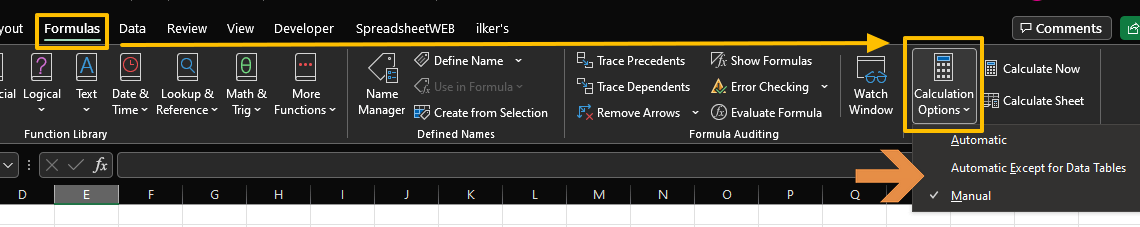Although manual calculation mode helps to deal with big files that take seconds or minutes to be calculated, waiting for the calculation of the entire workbook may not be convenient. Instead, it would be better to calculate certain cells for quick try-error changes. In this guide, we’re going to show you How to calculate selected cells only in Excel.

## Automatic vs. Manual Calculation

As you know, Excel's default calculation mode is automatic. This means that after each change in your workbook, Excel recalculates all changed cells and all dependent cells as well as volatile functions like OFFSET or INDIRECT. All these recalculations make your system suffer while calculating.

The alternative approach is; manual calculation. When you enable the manual calculation, Excel doesn't trigger calculations until you or a VBA code gives the command. Thus, you do not wait each time after updating a cell.

You can find the calculation mode in Calculations Options group under Formulas tab.Once the manual mode is active, you can use either commands from the same section or keyboard shortcuts to trigger the calculations.

 Calculate Now (in Ribbon) F9 Calculate Sheet (in Ribbon) Shift + F9 Calculate all worksheets in all workbooks Ctrl + Alt + F9 Full calculation with dependency tree rebuild Ctrl + Alt + Shift + F9

## Data

As an example, we have a column of formulas that should return the sum of the corresponding rows. On the other hand, the manual calculation is enabled in our Excel file and cells still show zero (0) values.Our intention is to calculate specific cells while others remain as zero.

## Calculating Selected Cells

Although it should already be clear, let us warn you beforehand that the calculation setting should be manual. If it is let's see the approaches.

## Single cells

Even in manual calculation mode, if you enter a formula to a cell and press Enter key, Excel will calculate the formula. Thus, there is no turning back if you are entering a new formula into a cell.### Non-VBA Approach

There is a weird technique to calculate the selected cells without VBA. All you need to do is to replace equal signs (=) with equal signs (=). Yes, you read correctly. Excel's Find & Replace feature allows you to edit multiple cells at once. This way, the previous single-cell trick can be applied to multiple cells. Here are the steps:

1. Select the cells you want to calculate.
2. Press Ctrl + H to open the Find & Replace
3. Enter an equal sign (=) to Find what and Replace with
4. Make sure the Within option is Sheet.
5. Click Replace All.6. You will see the informational dialog box with updated cells.### VBA Without Macro

Calculating selected cells is a single-line code in the VBA code.

`Selection.Calculate`

The good thing is that you can run this code in the Immediate Editor and save your workbook as an XLSM file.

1. Start by selecting cells you want to calculate
2. Open the VBA window by pressing Alt + F11.
3. Type or copy-paste the code into the Immediate
4. Press Enter key to run.Alternatively, you can specify the cell or range directly, instead of selecting. Use either Cells or Range objects instead of Selection.

```Cells(5,6).Calculate 'F5
Range("F6").Calculate 'F6
[F7:F9].Calculate 'F7:F9```

To learn more about referencing ranges in VBA, you can check out How to refer a range or a cell in Excel VBA article.

### Macro Approach

Alternatively, you can save the code in a macro and call it via shortcut or a Ribbon command.

1. Once the VBA window is open, insert a Module by using the Insert menu in the toolbar.
2. Copy&paste the code into the module you created.
3. Do not forget to save your file as an XLSM file to keep the macro in it.```Public Sub CalculateSelection()
If TypeName(Selection) = "Range" Then Selection.Calculate
End Sub```

To run the macro, click inside the macro to see the cursor in the Module window. Then either use the Play button in the toolbar or press F5 key.